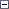## AtomineerUtils爆破过程记录

### AtomineerUtils爆破过程记录

AtomineerUtils是国外的一款用于生成源代码注释的一款VS插件，官方网站：http://www.atomineerutils.com/products.php```internal static bool b(bool A_0, bool A_1 = false)
{
bool flag = true;
int num = (((DateTime.Today.Year - 2010) * 365) + DateTime.Today.DayOfYear) << 8;
int num2 = 0;
try
{
num2 = Convert.ToInt32(c(f, "0", string.Empty));
}
catch
{
}
int num3 = (num - num2) >> 8;
if (num3 > 19)
{
if (e == 'I')
{
for (int i = 0; i < e.Length; i++)
{
StringBuilder builder = new StringBuilder();
for (int j = 0; j < e[i].Length; j++)
{
builder.Append((char) (e[i][j] ^ (16 | (j % 16))));
}
e[i] = builder.ToString();
}
}
if (num3 > 29)
{
flag = false;
Commands.f();
if ((num3 == g) || A_1)
{
return false;
}
MessageBox.Show(e + Environment.NewLine + Environment.NewLine + e + Environment.NewLine + Environment.NewLine + e, "Atomineer Pro Documentation", MessageBoxButtons.OK, MessageBoxIcon.Exclamation);
}
else
{
if ((num3 == g) || !A_0)
{
return true;
}
string[] strArray2 = new string[] { e, (30 - num3).ToString(), e, Environment.NewLine, Environment.NewLine, e };
if (MessageBox.Show(string.Concat(strArray2), "Atomineer Pro Documentation", MessageBoxButtons.YesNo, MessageBoxIcon.Exclamation) != DialogResult.Yes)
{
return true;
}
}
k.h("t~e}xzws");
g = num3;
}
return flag;
}```Collapse

```bool flag = true;
...
int num3 = (num2 - num2) >> 8;```

posted on 2017-02-22 12:39 FongLuo 阅读(276) 评论(0)  编辑 收藏 引用< 2020年9月 >
303112345
6789101112
13141516171819
20212223242526
27282930123
45678910

•

• 积分 - 46412
• 排名 - 416